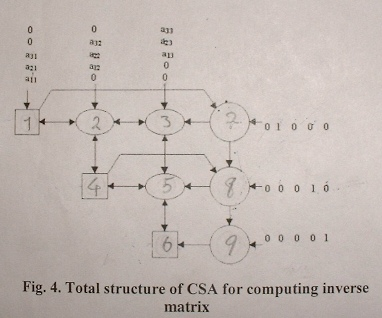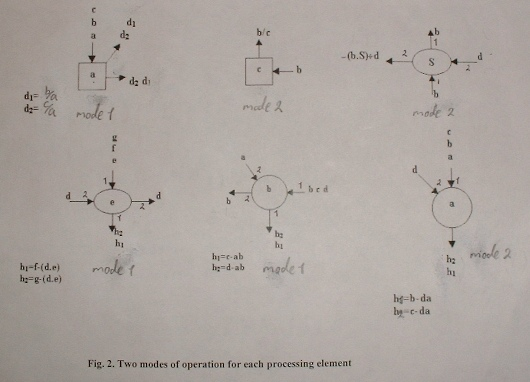Date Completed: September 03, 2003

The way that this array calculates the inverted matrix has been thoroughly studied in this paper: "Conical Systolic Array for Matrix Inversion" by H.S.Shahhoseini, A.Khayatzadeh, and M.Naderi (The paper). Herein, I explain the structure of the simulator program which was written by me with C++ language (2800+ LOC).

The mentioned conical systolic array (CSA) uses three types of processing elements (PEs). Each PE has been defined as an object in C++. For writing the program as modular as possible and taking advantage of virtual functions and also gathering the common functionalities of the PEs, a base class with the name of PE has been defined. The three types of PEs have been derived from this base class using public inheritance and the exclusive functionalities of each PE have been implemented in the respective class. These classes have been named according to their shapes in the paper as: EllipticPE, CircularPE, SquarePE. It should be mentioned that all four classes have been implemented regardless of the matrix dimension but due to some synchronization difficulties in one of the clock calculations, the main() function has some irregularities which prevents its generalization to any matrix dimension and in its current state, it is capable of matrix inversion calculation for a 3×3 matrix. The issue was under consideration of the authors of the paper but in the mean time, I was asked to conclude the project; in case that the problem is resolved, only the main() function will need modifications and the PEs' implementations won't need further changes.

At the beginning of the main() function, the CSA structure is created in memory using an array of SquarePE, an array of CircularPE, and a two-dimensional array of EllipticPE. Each PE knows its neighboring PEs using the embedded pointers. After receiving the matrix from user, the input matrix and the unity matrix elements are fed to the CSA in each clock according to the paper and calculation starts and continues. In each clock, first all PEs check their inputs and if there is any, they read and store them into their embedded input variables. Then calculations are performed on them and the results are stored in the embedded output variables so that they can be accessed in the next clock by other PEs. For realizing the mentioned procedure several flags have been defined such as: mode, outputUpdated, readyForCalculation, outputNumber,... and their names are descriptive of their role in the process. At the final clocks, the output elements are read and stored in a matrix and the inverted matrix is shown to the user. In each clock PEs' inputs and outputs and modes are shown step by step during the calculation as well.

The steps which are executed in the CSA for matrix inversion calculation:
Triangularization process:Inverted Matrix:The CSA Structure:
This structure is created in memory as described earlier. The number at the center of each shape designates the PE's number which is used to format the output of the program.Operation modes and input/output numbers of each PE type:This MS Excel file shows the inputs/outputs of each PE in every clock in parametric form:
CSA State Chart

The C++ source code and the executable file

CSA (Visual C++ 6.0 Project)